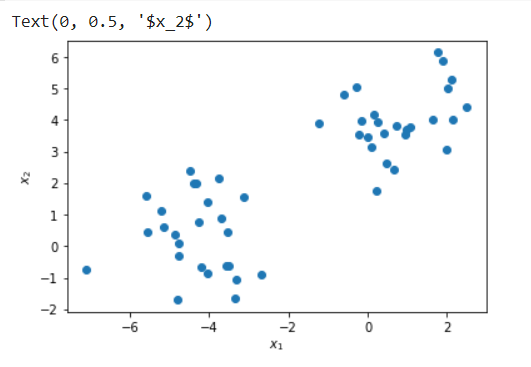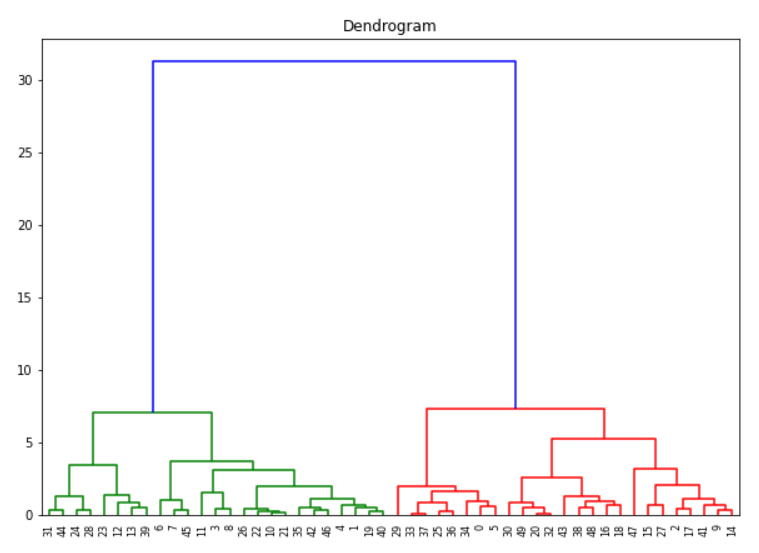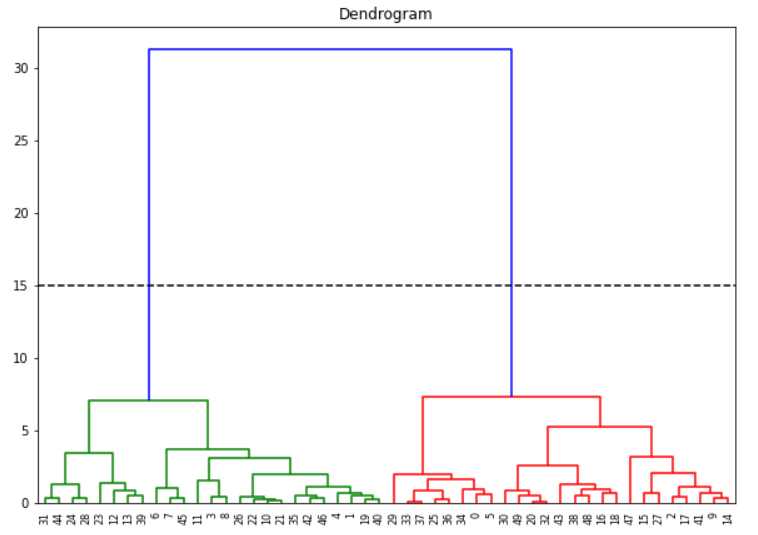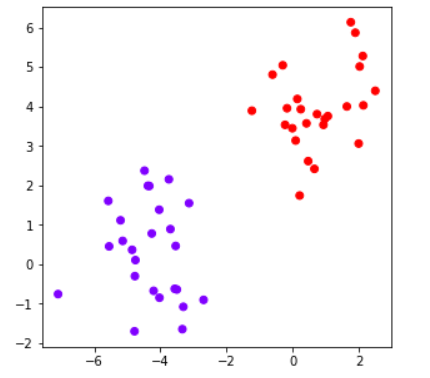# Agglomerative Hierarchical Clustering in Python Sklearn & Scipy```import pandas as pd
from sklearn.datasets import make_moons
import matplotlib.pyplot as plt
from sklearn.cluster import AgglomerativeClustering
from sklearn.datasets import make_blobs```

### The Dataset

Here we use make_blobs module of sklearn.datasets package of Scikit Learn to create a custom toy dataset of 50 data points with two features.

In :

```X, y = make_blobs(n_samples=50, centers=2, n_features=2,random_state=3)
df=pd.DataFrame(X,y)
df=df.rename(columns={0: "X1", 1:"X2"})

Out:

X1 X2
1 -3.336072 -1.644337
0 0.092166 3.139081
1 -5.552574 0.455115
0 -0.297907 5.047579
0 0.419308 3.574362

In :

```plt.scatter(X[:, 0], X[:, 1], label=y)
plt.xlabel("\$x_1\$")
plt.ylabel("\$x_2\$")```

Out:### Creating Dendrogram with Python Scipy

Python Scipy has dendrogram and linkage module inside scipy.cluster.hierarchy package that can be used for creating the dendrogram graph of agglomerative clustering.

Here we first create the linkage object with method = ward and affinity metric as euclidean and then use this to create the dendrogram.

In :

```#Dendrogram plot
plt.figure(figsize =(10,7))
plt.title('Dendrogram')

Out:### Determining No. of Clusters with Dendrogram

If you want to create flat clusters we can analyze the above dendrogram to determine no. of clusters. We first assume that the horizontal lines are extended on both sides, and as such, they would also cross the vertical lines. Now we have to identify the tallest vertical line that does not have any horizontal line crossing through it.

In the above dendrogram graph, such a vertical line is the blue line. We now draw a horizontal line across this vertical line as shown below. This horizontal line cuts the vertical line at two places, and this means the optimal number of clusters is 2.

Another way is to visually see which vertical line is showing the biggest jump. Since the vertical line denotes the distance or similarity between the two clusters, the big jump signifies the two clusters are not very similar. Again draw the horizontal line through this vertical line and the number of cuts it makes is optimal no. of clusters. Again in our example, it is the blue line, and the horizontal line cuts at two places so no. of clusters is 2.

(It should be noted however that these methods do not always guarantee the optimal number of clusters, it is just a guideline)In:

```cluster_ea = AgglomerativeClustering(n_clusters=2, linkage='ward',affinity='euclidean')

# Visualizing the clustering
plt.figure(figsize =(5, 5))
plt.scatter(df['X1'], df['X2'], c = cluster_ea.fit_predict(df),cmap='rainbow')
plt.show()```

Out: